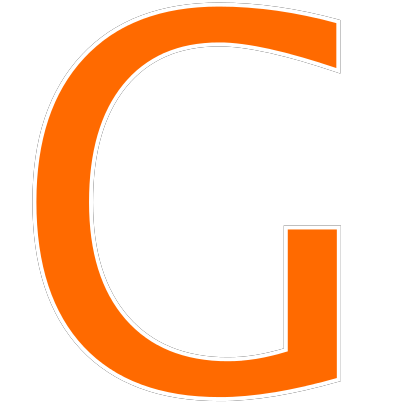Man Mask Weight Riddle, Man Mask Dumbbell Puzzle is the new puzzle trending on Facebook, Twitter & WhatsApp. In this Image Puzzle, you have to find out the value of weight + man x mask. This is a simple mathematics-based riddle in which we have to use the BODMAS rule to get the correct answer.

So today in this post we are going to solve this Man Mask Weight Riddle and provide the correct Man Mask Weight Puzzle answer. Just scroll down to get the answer and solution for the puzzle.

## Man Mask Weight Riddle Solution

Let’s find the Man Mask Weight Riddle solution.

First, we have to find the value of each man. So Man = 15/3 = 5

Now the value of Mask = 9/3 = 3

Whereas Weight = 18/3 = 6

So 1 Weight Plate = 6/6=1

Finally, now we have the value of Man, Mask, Weight, and Weight Plate. So we can find a solution.

The final equation is:-

Weights 2 pair with 4 plates each + Man with mask and Weight with 4 plates x one mask

= (4+4) + (5+3+4) x 3

= 8 + 12 x 3 = 44

So the correct answer to the Man Mask Weight Riddle Puzzle is 44